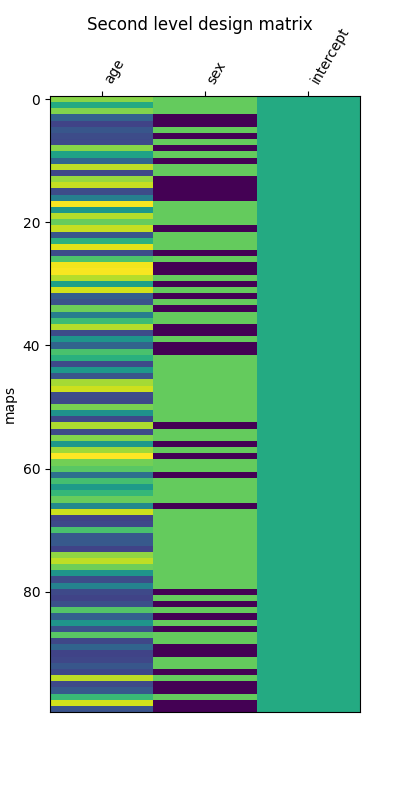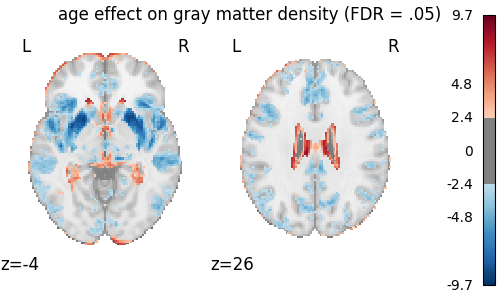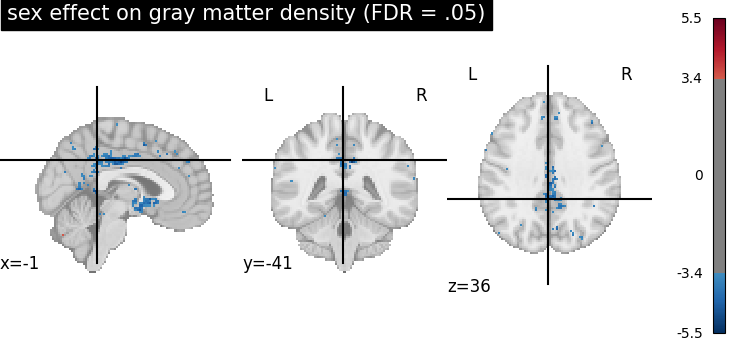# Voxel-Based Morphometry on OASIS dataset#

This example uses voxel-based morphometry (VBM) to study the relationship between aging, sex, and gray matter density.

The data come from the OASIS project. If you use it, you need to agree with the data usage agreement available on the website.

It has been run through a standard VBM pipeline (using SPM8 and NewSegment) to create VBM maps, which we study here.

## VBM analysis of aging#

We run a standard GLM analysis to study the association between age and gray matter density from the VBM data. We use only 100 subjects from the OASIS dataset to limit the memory usage.

Note that more power would be obtained from using a larger sample of subjects.

```n_subjects = 100  # more subjects requires more memory
```

```from nilearn import datasets

oasis_dataset = datasets.fetch_oasis_vbm(
n_subjects=n_subjects,
legacy_format=False,
)
gray_matter_map_filenames = oasis_dataset.gray_matter_maps
age = oasis_dataset.ext_vars["age"].astype(float)
```

Sex is encoded as ‘M’ or ‘F’. Hence, we make it a binary variable.

```sex = oasis_dataset.ext_vars["mf"] == "F"
```

Print basic information on the dataset.

```print(
"First gray-matter anatomy image (3D) is located at: "
f"{oasis_dataset.gray_matter_maps}"
)
print(
"First white-matter anatomy image (3D) is located at: "
f"{oasis_dataset.white_matter_maps}"
)
```
```First gray-matter anatomy image (3D) is located at: /home/remi/nilearn_data/oasis1/OAS1_0001_MR1/mwrc1OAS1_0001_MR1_mpr_anon_fslswapdim_bet.nii.gz
First white-matter anatomy image (3D) is located at: /home/remi/nilearn_data/oasis1/OAS1_0001_MR1/mwrc2OAS1_0001_MR1_mpr_anon_fslswapdim_bet.nii.gz
```

Get a mask image: A mask of the cortex of the ICBM template.

```gm_mask = datasets.fetch_icbm152_brain_gm_mask()
```

```from nilearn.image import resample_to_img

gray_matter_map_filenames,
interpolation="nearest",
)
```

## Analyse data#

First, we create an adequate design matrix with three columns: ‘age’, ‘sex’, and ‘intercept’.

```import numpy as np
import pandas as pd

intercept = np.ones(n_subjects)
design_matrix = pd.DataFrame(
np.vstack((age, sex, intercept)).T,
columns=["age", "sex", "intercept"],
)
```

Let’s plot the design matrix.

```from nilearn import plotting

ax = plotting.plot_design_matrix(design_matrix)
ax.set_title("Second level design matrix", fontsize=12)
ax.set_ylabel("maps")
``````Text(29.000000000000007, 0.5, 'maps')
```

Next, we specify and fit the second-level model when loading the data and also smooth a little bit to improve statistical behavior.

```from nilearn.glm.second_level import SecondLevelModel

second_level_model.fit(
gray_matter_map_filenames,
design_matrix=design_matrix,
)
```
```SecondLevelModel(mask_img=<nibabel.nifti1.Nifti1Image object at 0x7fd1efe8dc10>,
smoothing_fwhm=2.0)```
In a Jupyter environment, please rerun this cell to show the HTML representation or trust the notebook.

Estimating the contrast is very simple. We can just provide the column name of the design matrix.

```z_map = second_level_model.compute_contrast(
second_level_contrast=[1, 0, 0],
output_type="z_score",
)
```

We threshold the second level contrast at FDR-corrected p < 0.05 and plot it.

```from nilearn.glm import threshold_stats_img

_, threshold = threshold_stats_img(z_map, alpha=0.05, height_control="fdr")
print(f"The FDR=.05-corrected threshold is: {threshold:03g}")

display = plotting.plot_stat_map(
z_map,
threshold=threshold,
colorbar=True,
display_mode="z",
cut_coords=[-4, 26],
title="age effect on gray matter density (FDR = .05)",
)
plotting.show()
``````The FDR=.05-corrected threshold is: 2.40175
```

We can also study the effect of sex by computing the contrast, thresholding it and plot the resulting map.

```z_map = second_level_model.compute_contrast(
second_level_contrast="sex",
output_type="z_score",
)
_, threshold = threshold_stats_img(z_map, alpha=0.05, height_control="fdr")
plotting.plot_stat_map(
z_map,
threshold=threshold,
colorbar=True,
title="sex effect on gray matter density (FDR = .05)",
)
``````<nilearn.plotting.displays._slicers.OrthoSlicer object at 0x7fd1efce5d90>
```

Note that there does not seem to be any significant effect of sex on gray matter density on that dataset.

## Generating a report#

It can be useful to quickly generate a portable, ready-to-view report with most of the pertinent information. This is easy to do if you have a fitted model and the list of contrasts, which we do here.

```from nilearn.reporting import make_glm_report

icbm152_2009 = datasets.fetch_icbm152_2009()
report = make_glm_report(
model=second_level_model,
contrasts=["age", "sex"],
bg_img=icbm152_2009["t1"],
)
```

We have several ways to access the report:

```# report  # This report can be viewed in a notebook
# report.save_as_html('report.html')
# report.open_in_browser()
```

Total running time of the script: (2 minutes 15.173 seconds)

Estimated memory usage: 1478 MB

Gallery generated by Sphinx-Gallery